# Olympiad Test: Embedded Figures - 1

## 10 Questions MCQ Test National Cyber Olympiad Class 8 | Olympiad Test: Embedded Figures - 1

Description
Attempt Olympiad Test: Embedded Figures - 1 | 10 questions in 20 minutes | Mock test for Class 8 preparation | Free important questions MCQ to study National Cyber Olympiad Class 8 for Class 8 Exam | Download free PDF with solutions
QUESTION: 1

Solution:
QUESTION: 2

Solution:
QUESTION: 3

### Find out the alternative figure which contains figure (X) as its part.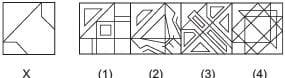Solution:
QUESTION: 4

Find out the alternative figure which contains figure (X) as its part.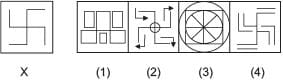Solution:
QUESTION: 5

Find out the alternative figure which contains figure (X) as its part.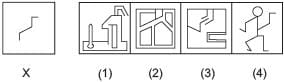Solution:
QUESTION: 6

Find out the alternative figure which contains figure (X) as its part.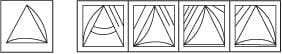Solution:
QUESTION: 7

Find out the alternative figure which contains figure (X) as its part.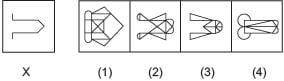Solution:
QUESTION: 8

Find out the alternative figure which contains figure (X) as its part.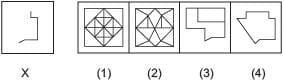Solution:
QUESTION: 9

Find out the alternative figure which contains figure (X) as its part.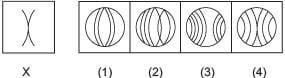Solution:
QUESTION: 10

Find out the alternative figure which contains figure (X) as its part.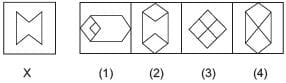Solution:Use Code STAYHOME200 and get INR 200 additional OFF Use Coupon Code